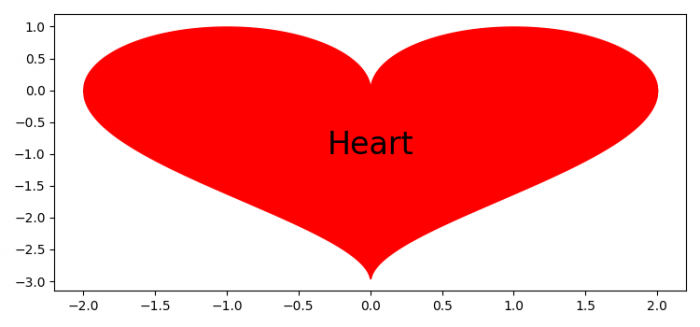# How to draw a heart with pylab?

To draw a heart with pylab/pyplot, we can follow the steps given below −

## Steps

• Set the figure size and adjust the padding between and around the subplots.
• Create x, y1 and y2 data points using numpy.
• Fill the area between (x, y1) and (x, y2) using fill_between() method.
• Place text on the plot using text() method at (0, -1.0) point.
• To display the figure, use show() method.

## Example

import numpy as np
from matplotlib import pyplot as plt

plt.rcParams["figure.figsize"] = [7.50, 3.50]
plt.rcParams["figure.autolayout"] = True

x = np.linspace(-2, 2, 1000)
y1 = np.sqrt(1 - (abs(x) - 1) ** 2)
y2 = -3 * np.sqrt(1 - (abs(x) / 2) ** 0.5)

plt.fill_between(x, y1, color='red')
plt.fill_between(x, y2, color='red')

plt.text(0, -1.0, 'Heart', fontsize=24, color='black',
horizontalalignment='center')

plt.show()

## Output